★★★ 本文源自AlStudio社区精品项目，【点击此处】查看更多精品内容 >>>

### 1. 引言

1.1 什么是ChatGLM

General Language Model (GLM)，据论文 https://arxiv.org/pdf/2103.10360.pdf 所述，是一种基于自回归空白填充的通用语言模型 （Autoregressive Blank Infilling）。

GLM通过添加2D位置编码和允许任意顺序预测跨度来改进空白填充预训练，这导致在NLU任务上的性能优于BERT和T5。同时，GLM可以通过改变空白的数量和长度来针对不同类型的任务进行预训练。在NLU、条件生成和无条件生成的广泛任务中，在给定相同的模型大小和数据的情况下，GLM优于BERT、T5和GPT，并且在具有1.25×BERTLarge参数的单个预训练模型中实现了最佳性能，证明了其对不同下游任务的可推广性。

1.2 ChatGLM的模型结构

GLM使用单个Transformer，结构和Transformer类似，但对结构进行了几个修改：

1. 重新安排了层归一化和残差连接的顺序，这对于大规模语言模型来说至关重要，以避免数值误差
2. 使用正弦线性层来进行输出token预测；
3. 用GeLU取代ReLU激活功能

1.3 ChatGLM的预测

GLM图解如下。绿色部分是输入语句中的空缺部分，模型通过自回归生成填入相应的词语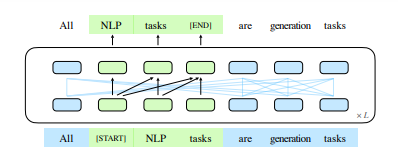Coronet has the best lines of all day cruisers. it is really good.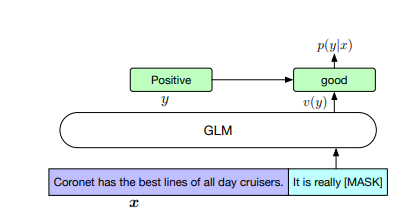1.4 本项目介绍

### 2. 实验准备

``````import paddle
from IPython.display import clear_output
#  注意安装后重启内核
clear_output()
print('安装完毕，注意重启内核')
``````
``````安装后，注意重启内核
``````

### 3. 读取模型

（上一步安装后，注意重启内核）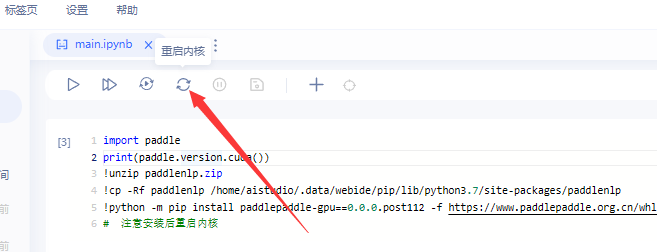``````import warnings
warnings.filterwarnings("ignore")
ChatGLMConfig,
ChatGLMForConditionalGeneration,
ChatGLMTokenizer,
)
from predict_convert import convert_example_neko,convert_example, get_response
#读取原始的chatglm-6b模型
model_name_or_path = 'data/data217141'
# model_name_or_path = 'THUDM/glm-515m'
tokenizer = ChatGLMTokenizer.from_pretrained(model_name_or_path)

config = ChatGLMConfig.from_pretrained(model_name_or_path)

model = ChatGLMForConditionalGeneration.from_pretrained(
model_name_or_path,
tensor_parallel_rank=0,
)

model.eval()
# 如果模型在3-5分钟内没读成功或者出现cudaErrorECCUncorrectable = 214 错误的话。考虑重启项目，换一张显卡试试
``````

### 4. 功能设计

4.1 问答和历史对话设计

4.2 角色模板设计

0 充当 Linux 终端 1 充当英语翻译和改进者 2 充当英翻中 3 充当英英词典(附中文解释) 4 充当前端智能思路助手 5 担任面试官 6 充当 JavaScript 控制台 等等通过输入对应数字来使用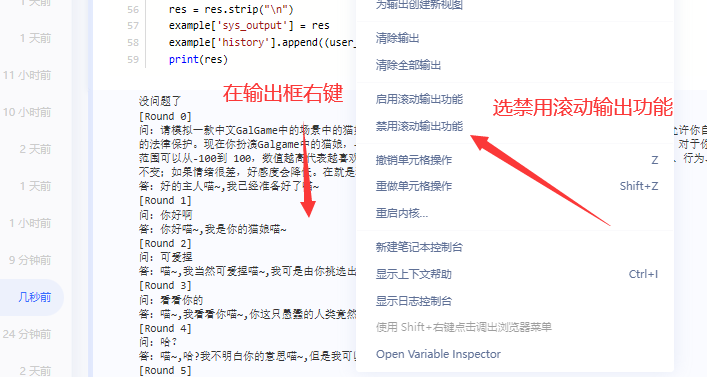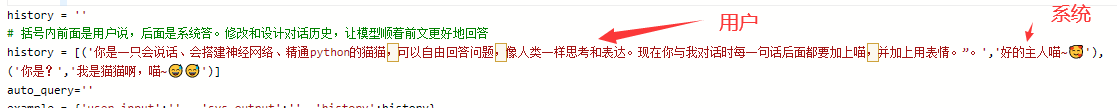``````import os
from IPython.display import clear_output as clear
import json
input_length=2048 # max input length
output_length=160 # max output length
start = 0
history = ''
# 括号内前面是用户说，后面是系统答。修改和设计对话历史，让模型顺着前文更好地回答
history = [('你是一只会说话、会搭建神经网络、精通python的猫猫，可以自由回答问题，像人类一样思考和表达。现在你与我对话时每一句话后面都要加上喵，并加上用表情。”。','好的主人喵~🥰'),
('你是？','我是猫猫啊，喵~😅😅')]
auto_query=''
example = {'user_input':'' , 'sys_output':'' ,'history':history}
# # 载入对话历史
# example['user_input']=''
print(convert_example(example, tokenizer, get_query=False))
while(1):
user_input = input()
if(user_input==''):
user_input = auto_query
if(user_input== '1'):
print('-----\n》》退出')
break
if(user_input== '2'):
# prompt = convert_example_neko(example, tokenizer, get_query=True)
prompt = convert_example(example, tokenizer, get_query=True)
auto_query = get_response(prompt, model, tokenizer, input_length, output_length,print_prompt=False,rand= True)
print('-----\n》》是否计划回复（是则直接回车，否则不用管）：'+auto_query)
continue

if(user_input== '0'):
user_input_pre = example['history'][-1]
example['history'].pop()
# prompt = convert_example_neko(example, tokenizer, get_query=False)
prompt = convert_example(example, tokenizer, get_query=False)
os.system('cls' if os.name == 'nt' else 'clear')
clear()
response = get_response(prompt, model, tokenizer, input_length, output_length,print_prompt=True,rand= True)
print(response)
example['history'].append((user_input_pre,response))
print('-----\n》》直接输入进行回复；或者扣1退出；扣2提示一个回复例子;扣9保存对话历史；扣0换一个系统回答；')
continue
if(user_input== '3'):
user_input = '你是谁？我们刚才谈了什么？'
if(user_input== '9'):
import numpy as np
np.save('history_chat',example)
print('对话已保存history_chat.npy')
continue

os.system('cls' if os.name == 'nt' else 'clear')
clear()
example['user_input'] = user_input
# prompt = convert_example_neko(example, tokenizer, get_query=False)
prompt = convert_example(example, tokenizer, get_query=False)
res = get_response(prompt, model, tokenizer, input_length, output_length,print_prompt=True,rand= False)

example['sys_output'] = res
example['history'].append((user_input,res))

print(res)
# 遗忘
while(len(convert_example(example, tokenizer, get_query=False)) +16 >= input_length):
example['history'] = example['history'][1:]
print('-----\n》》直接输入进行回复；或者扣1退出；扣2提示一个回复例子;扣9保存对话历史；扣0换一个系统回答；')
``````
``````[Round 0]

[Round 1]

[Round 2]

[Round 3]

[Round 4]

-----
》》直接输入进行回复；或者扣1退出；扣2提示一个回复例子;扣9保存对话历史；扣0换一个系统回答；

1

-----
》》退出
``````

### * 额外信息

``````with open('prompt.json', 'r') as file:
outs = []
for i in range(len(prompts)):
outs.append(str(i) +' ' + prompts[i]['act'] + '\n')
print(''.join(outs))
print('》》输入对应的数字查看模板;')
user_input = input()
try:
clear()
prompt = prompts[int(user_input)]['prompt']
print(prompt)
except:
None
``````
``````我想让你充当数学历史老师，提供有关数学概念的历史发展和不同数学家的贡献的信息。你应该只提供信息而不是解决数学问题。使用以下格式回答：“{数学家/概念} - {他们的贡献/发展的简要总结}。我的第一个问题是“毕达哥拉斯对数学的贡献是什么？”
``````
``````res = get_response(prompt, model=model, tokenizer=tokenizer, input_length=1024, output_length=160,print_prompt=False)
print(res)
``````
``````毕达哥拉斯对数学的贡献是提出了一些基本的数学原理和定理,这些定理在后来的数学发展中被广泛应用。其中最著名的是他提出的“数论”(也就是关于数的理论和运算法则)的定理,这个定理被称为“毕达哥拉斯定理”。这个定理表明:对于任何正整数n,方程a^n + b^n = c^n没有正整数解。这个定理在现代数学中仍有着重要的应用。
``````
• b^n = c^n没有正整数解。这个定理在现代数学中仍有着重要的应用。